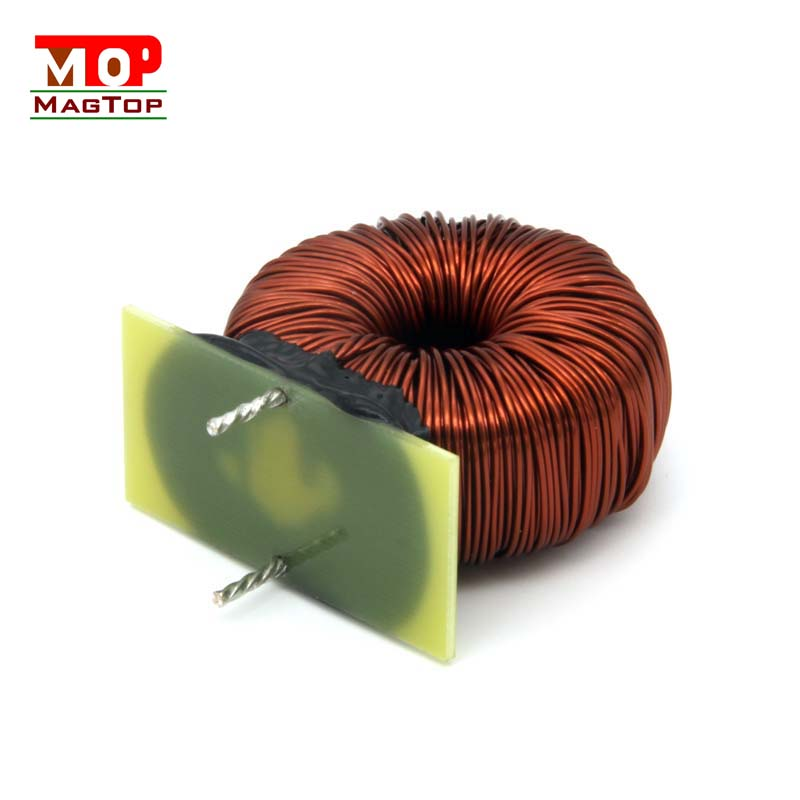# What's the difference between a magnetic bead and an inductor? Analysis of 6 differences between inductors and magnetic beads

##### author: MagTop
06/01/2023Power Inductor and magnetic beads are similar in appearance and function. Many people think that they are "straight apart from traffic", so that many people confuse the two.
In fact, whether principle or application, inductance and magnetic beads are not small differences.

1. The magnetic material of inductance is open, and part of the magnetic force line passes through the magnetic core and part through the air.

While the magnetic material of the magnetic bead is closed, almost all the magnetic force lines are closed in the magnetic ring, which is cleaner.

2. The unit of inductance is the inductance value (H), and the unit of magnetic bead is the impedance (ohm), which is generally the impedance value of 100Mhz.

One important thing is that even if the parameters of the same magnetic beads, their filtering performance will be very different.

Because the magnetic bead parameter is labeled with the impedance of a specific frequency point (120Mhz), even if the impedance of this frequency point is the same, the impedance of other frequency points will be very different.

3. The impedance of the magnetic bead is the result of the joint action of reactance X and resistance R, which is dominated by X at low frequency and R at high frequency. The inductor is mostly used in the low frequency band (50Mhz), while the magnetic bead is mostly used in the high-frequency filtering scene, where R will absorb noise and convert into heat, so the unit of the magnetic bead is ohm.

4. The filter principle of inductance is to convert electric energy into magnetic energy, and then convert the magnetic energy back into electric energy (noise) or radiation (EMI). The magnetic bead is to convert the electric energy into heat energy, and the magnetic bead is a more "clean" filter element.

5. Inductance is an energy storage element, which may be self-excited with capacitance during filtering;
The magnetic bead is an energy dissipating element (R), and does not self-excite when working with the capacitor.
6, inductance and magnetic beads have rated current parameters, the difference is that when the current exceeds the rated current, the effective inductance will decline, and magnetic beads may be burned directly.

In a word, the Wound inductor works in the frequency band where the reactance is much greater than the resistance. At this time, the reactance is mainly inductive, whose unit is inductance H.

Because the reactance component is the main component, the component is very small, so the inductance is mainly the role of energy storage.

The magnetic bead mainly works in the frequency band where the resistance is greater than the reactance, and the resistance is the main component, so the unit of the magnetic bead is ohms.